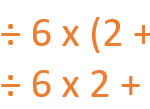Smartick is a fun way to learn math!# I want to learn about: Combined Operations

Learn about combined operations with Smartick. Activities, exercises, tutorials, and problems with combined operations for elementary aged children. Here you will find posts about combined operations to make learning mathematics easier and more fun. Aprende combined operations con Smartick.

Feb03

## Working on Combined Operations through Word ProblemsIn today’s post, we are going to present the way that we work on combined operations through word problems in Smartick. In the field of mathematics in school, combined operations are those problems where we need to use more than one operation. If you don’t remember exactly what they were, click here to refresh your memory! […]

Nov21

## Combined Operations: How to Solve These Types of ProblemsCombined operations In this post, we are going to learn the order of operations that you have to follow to do combined operations: addition, subtraction, multiplication, and division. Combined operations cannot be done in a random manner. You have to follow an order: Step 1: Do the operations that are inside of the parentheses. For […]

Nov09

## How to Solve Combined OperationsIn this post, we will learn the order that needs to be followed to solve combined operations: addition, subtraction, multiplication, and division. The combined operations cannot be performed at random, an order must be followed. Step 1: Perform operations that are within parenthesis. For example: 3 x (2 + 4). First, we do the operation […]

Oct14

## What Are Combined Operations?

What are combined operations? They are those in which various arithmetic operations appear: adding, subtracting, multiplying and dividing. How do you solve them? To solve combined operations correctly you have to follow the following steps: STEP 1: Do the operations that are inside of the parentheses. In our example, we have inside of the parentheses […]

Sep12

## Tricks to Solve Combined Operations

In today’s post we will learn some tricks to solve combined operations in a simpler way. Example 1 Look at these two operations: Have you finished it yet? Have you noticed that the two results are the same? Which of the two was easier to multiply? Converting an operation to an equivalent one can help […]